Working with E8+++

I wanted to confirm some work being done with E11 (or more specifically the Extended E8+++), so I used my “VisibLie” notebook (which includes the “SuperLie” package for analyzing Lie Algebras) to get the following information:

I first created the Dynkin diagram which produces the Cartan Matrix: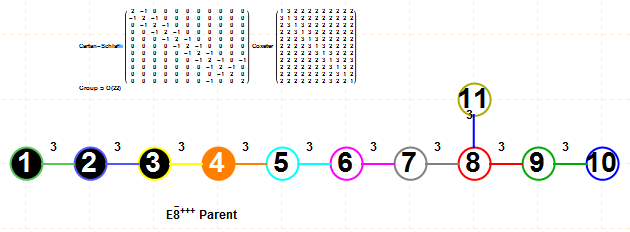This allows the evaluation of the EigenSystem of the Cartan matrix as follows: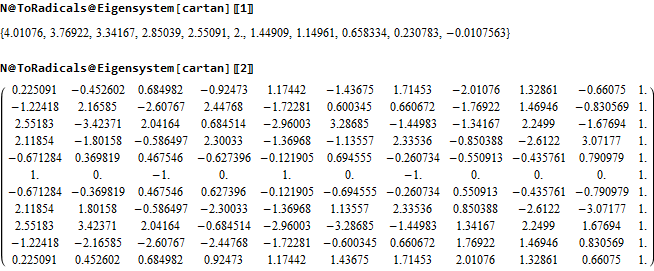As well as using the SuperLie package to evaluate the Positive Roots, Weights and Heights of the E8+++ (The first 80 are shown. For the full list in .pdf click here or click on the image below):The 4870 positive roots up to height 47 generate a Hasse diagram as follows (with 3 sections zoomed in):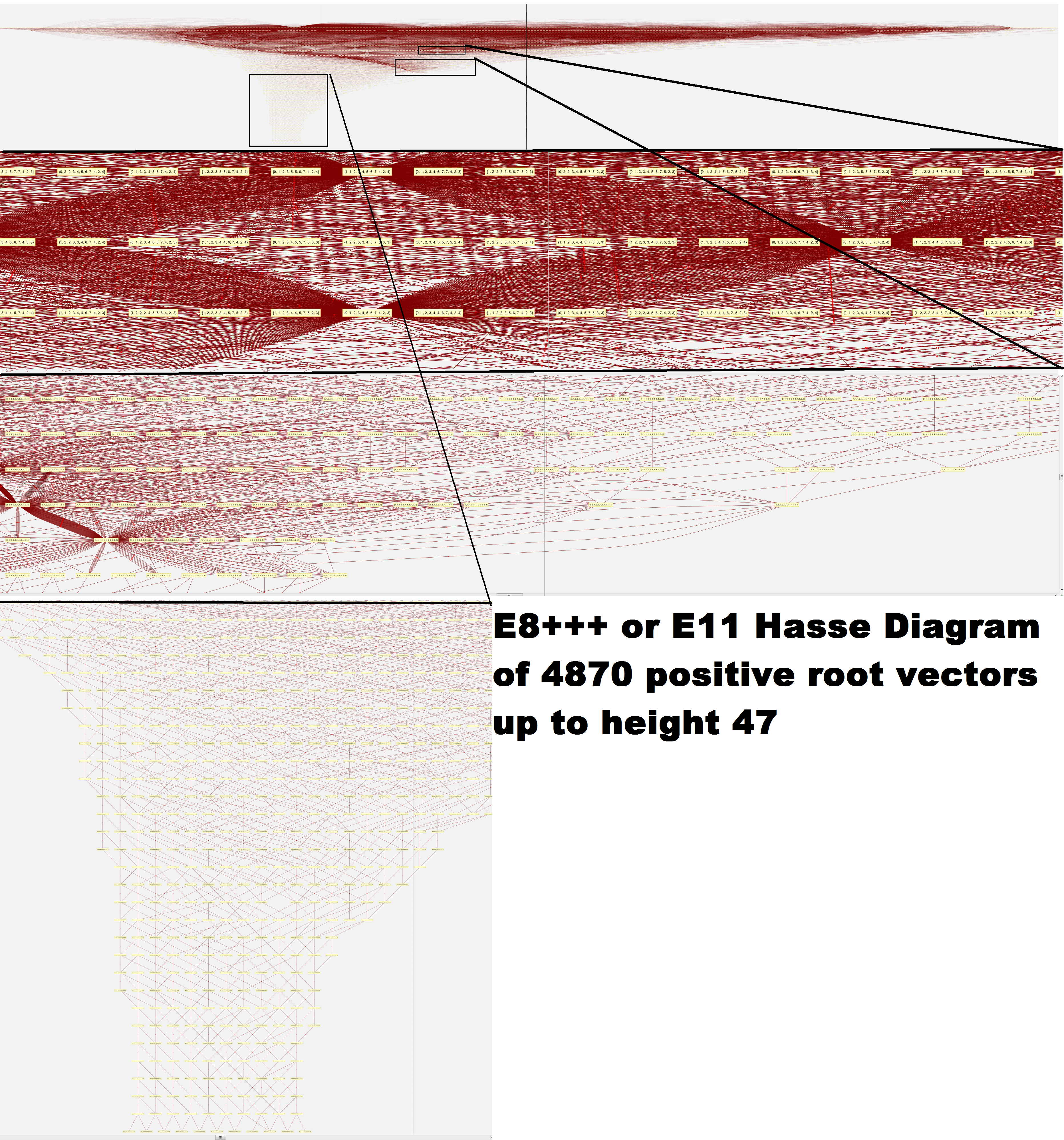I am working on replication of the Petrie projection by A.G. Lisi based on his prescription using his basis vectors for 2D projection and a Simple Roots Matrix: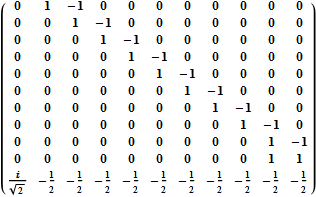Adjusting this E11 Simple Roots Matrix (srmE11) to match the Dynkin diagram above and then verifying it creates the same Cartan matrix, we have: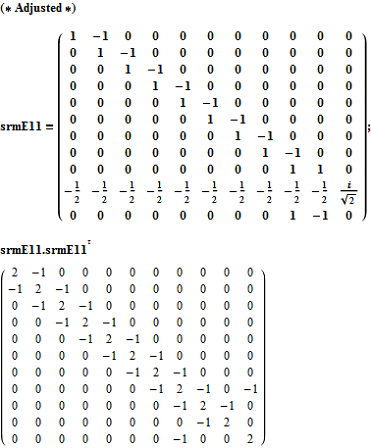To get the 9740 E8+++ vertices, we simply use the dot product of the transpose of srmE11 against the positive and negative root vectors (first 50 shown):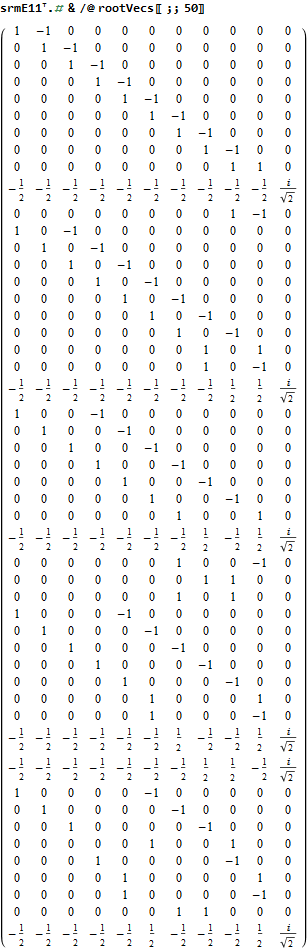A first cut at plotting these involves projecting them to a 2D using the dot product of the 2 basis vectors provided, which gives the following ListPlot: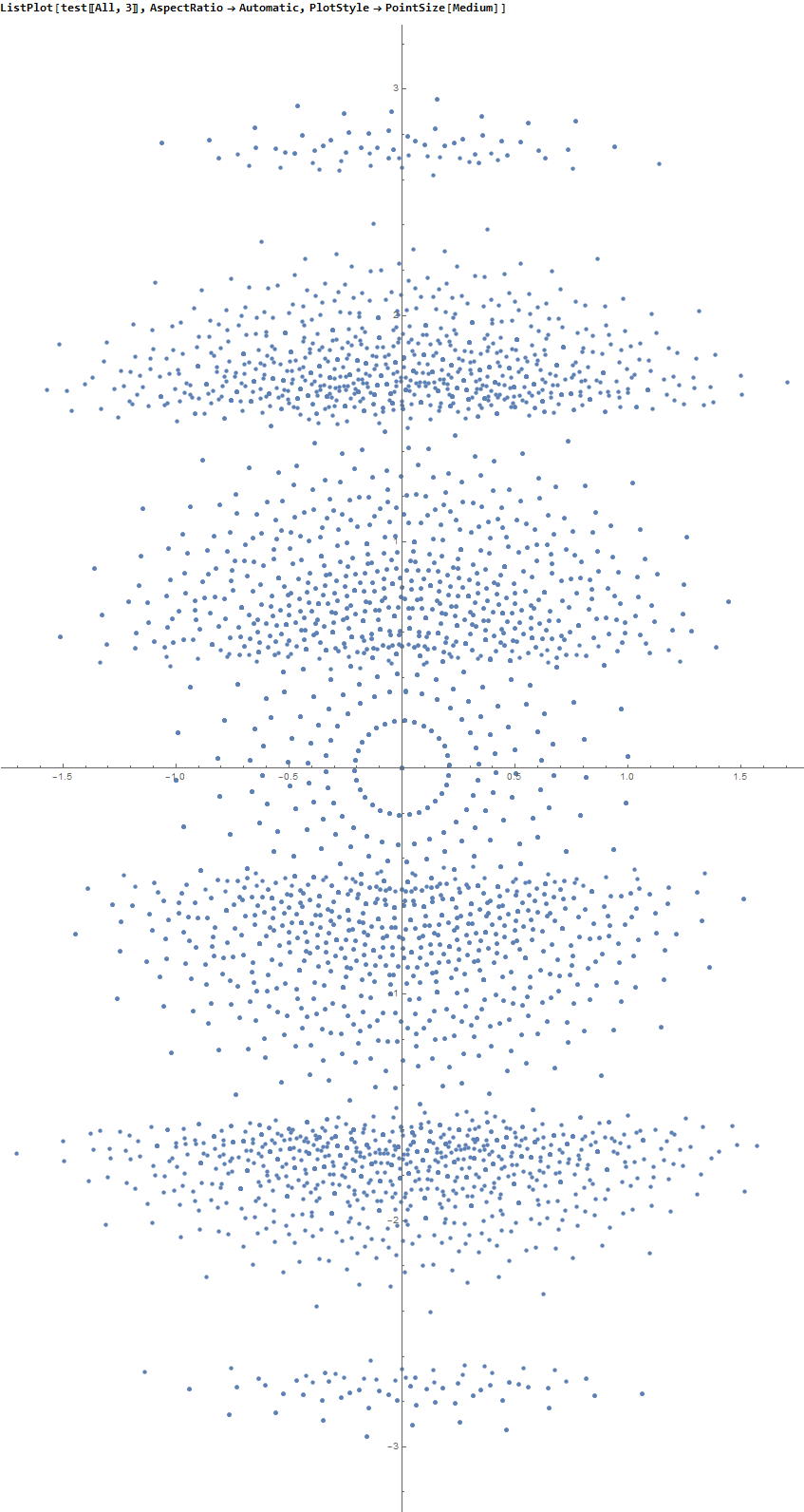The limited results from SuperLie are consistent with the image given by Lisi on the Bee’s BackReaction blog, after eliminating the vertical “levels” in my raw plot.

Email me if you want more detail, see errors, or would like to help.

Greg
JGMoxness@theoryofeverything.org

Mapping the fourfold H4 600-cells emerging from E8: A mathematical and visual study

My latest paper is now available here or on Vixra.

{abstract}
It is widely known that the E8 polytope can be folded into two Golden Ratio (Φ) scaled copies of the 4 dimensional (4D) 120 vertex 720 edge H4 600-cell. While folding an 8D object into a 4D one is done by applying the dot product of each vertex to a 4×8 folding matrix, we use an 8×8 rotation matrix to produce four 4D copies of H4 600-cells, with the original two left side scaled 4D copies related to the two right side 4D copies in a very specific way. This paper will describe and visualize in detail the specific symmetry relationships which emerge from that rotation of E8 and the emergent fourfold copies of H4. It will also introduce a projection basis using the Icosahedron found within the 8×8 rotation matrix. It will complete the detail for constructing E8 from the 3D Platonic solids, Icosians, and the 4D H4 600-cell. Eight pairs of Φ scaled concentric Platonic solids are identified directly using the sorted and grouped 3D projected vertex norms present within E8. Finally, we will show the relationship of the Beordijk-Coxeter Tetrahelix emerging from the Petrie projection’s concentric rings of 30 vertices of the H4 600-cells.

This paper builds off of some recent work here, and here.

Below are a few figures from that paper.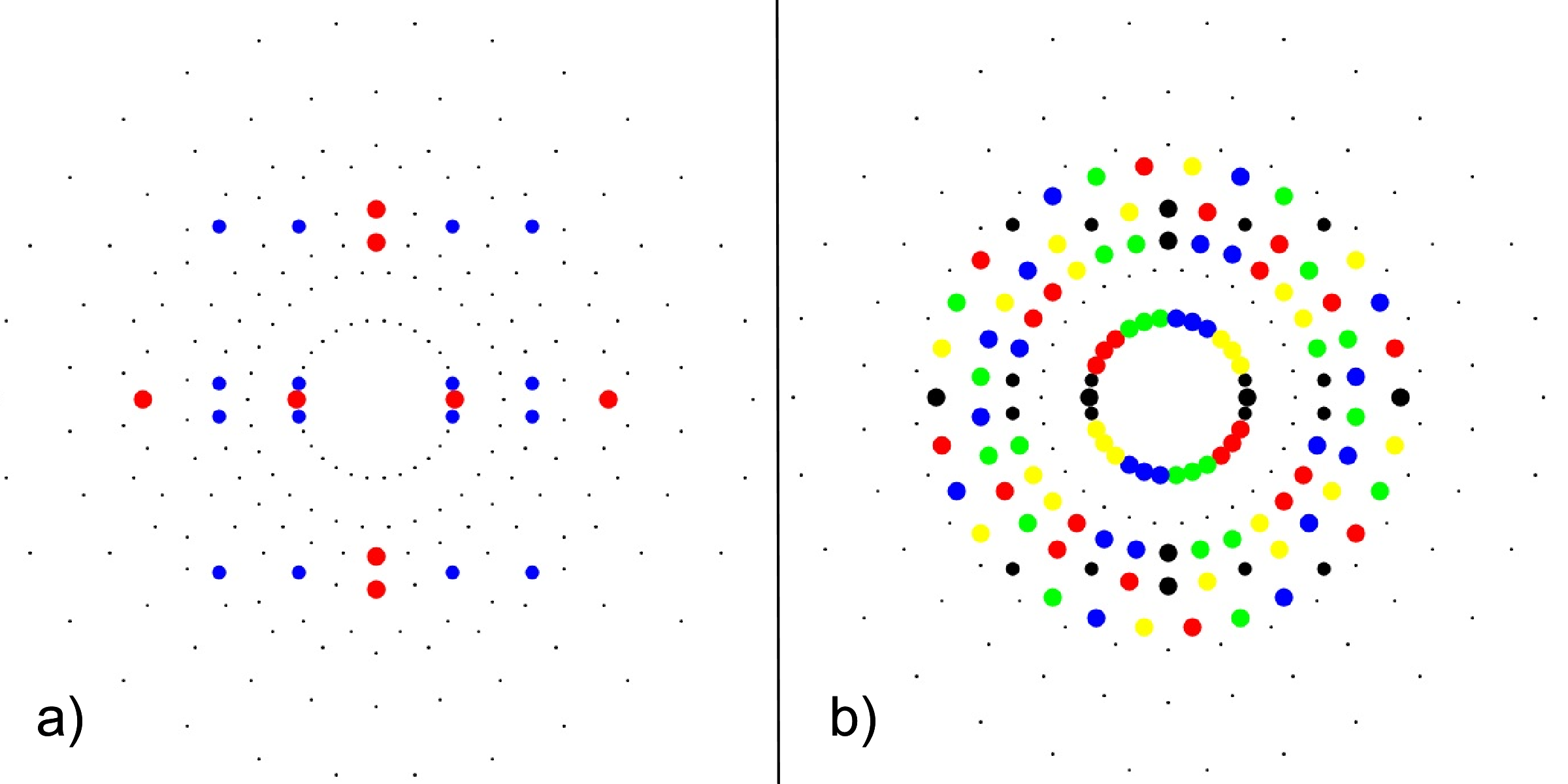FIG. 3: a) 24-cell highlighting the 16-cell (red on-axis vertices) and 8-cell (blue off-axis vertices), b) Snub 24-cell highlighting four pi/5 rotations of the 24-cell (black) in red, green, blue, yellow.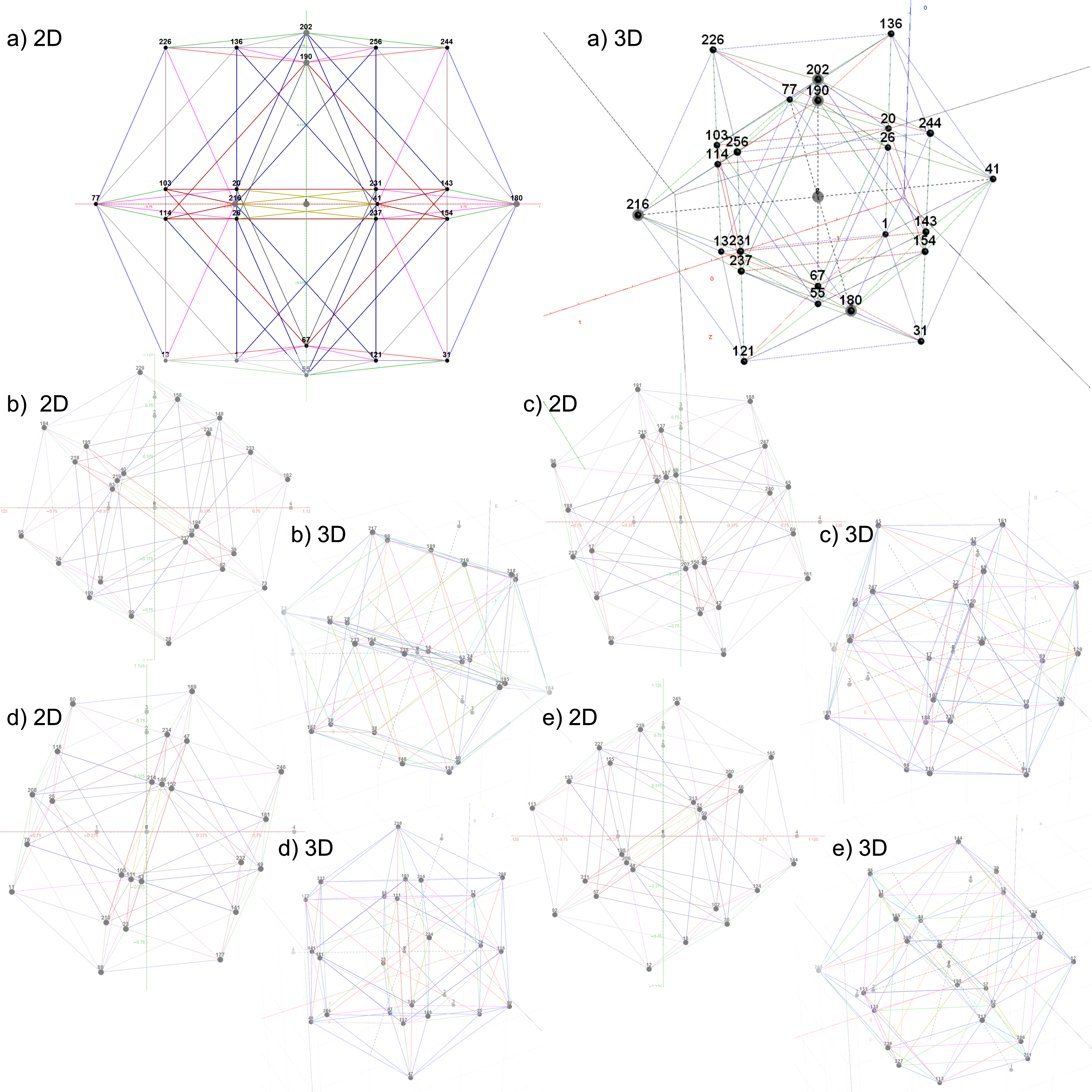FIG. 4: The 5 24-cell decomposition of one 600-cell, each with 2D and 3D vertex numbered projections including edges; a)
24-cell, b-e) Snub 24-cells from four pi/5 rotations of the 24-cell in a)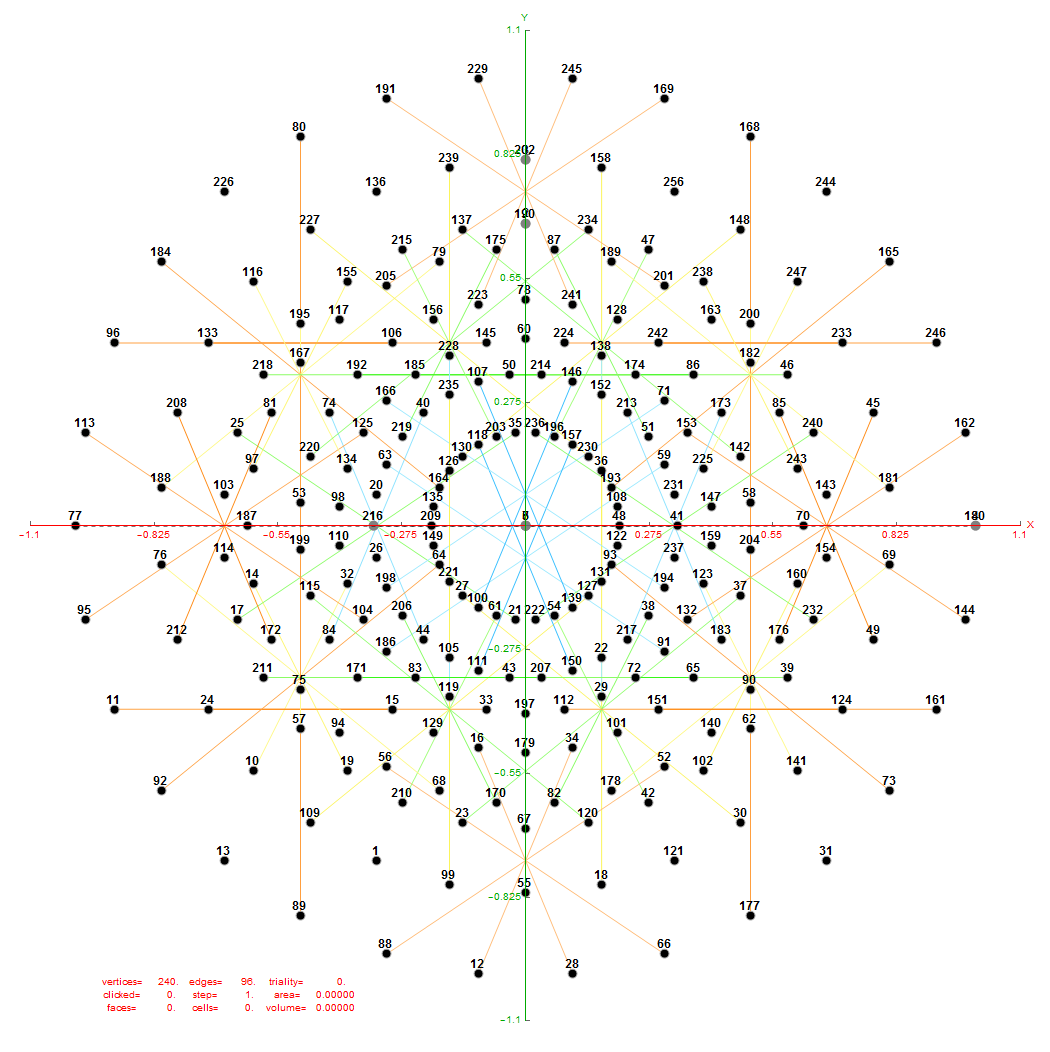FIG. 6: Vertex numbered Petrie projection of the rotated E8, showing the 96 edges of 8D norm l = 2Φ which links the Snub 24-cell H4 and H4Φ (L<->R) vertices (Note: the 8 excluded positive E8 8-Orthoplex (or equivalently, the H4 and H4Φ 4-Orthoplex) “generator vertices” are shown as larger gray labeled axis dots which overlap their darker black E8 vertices)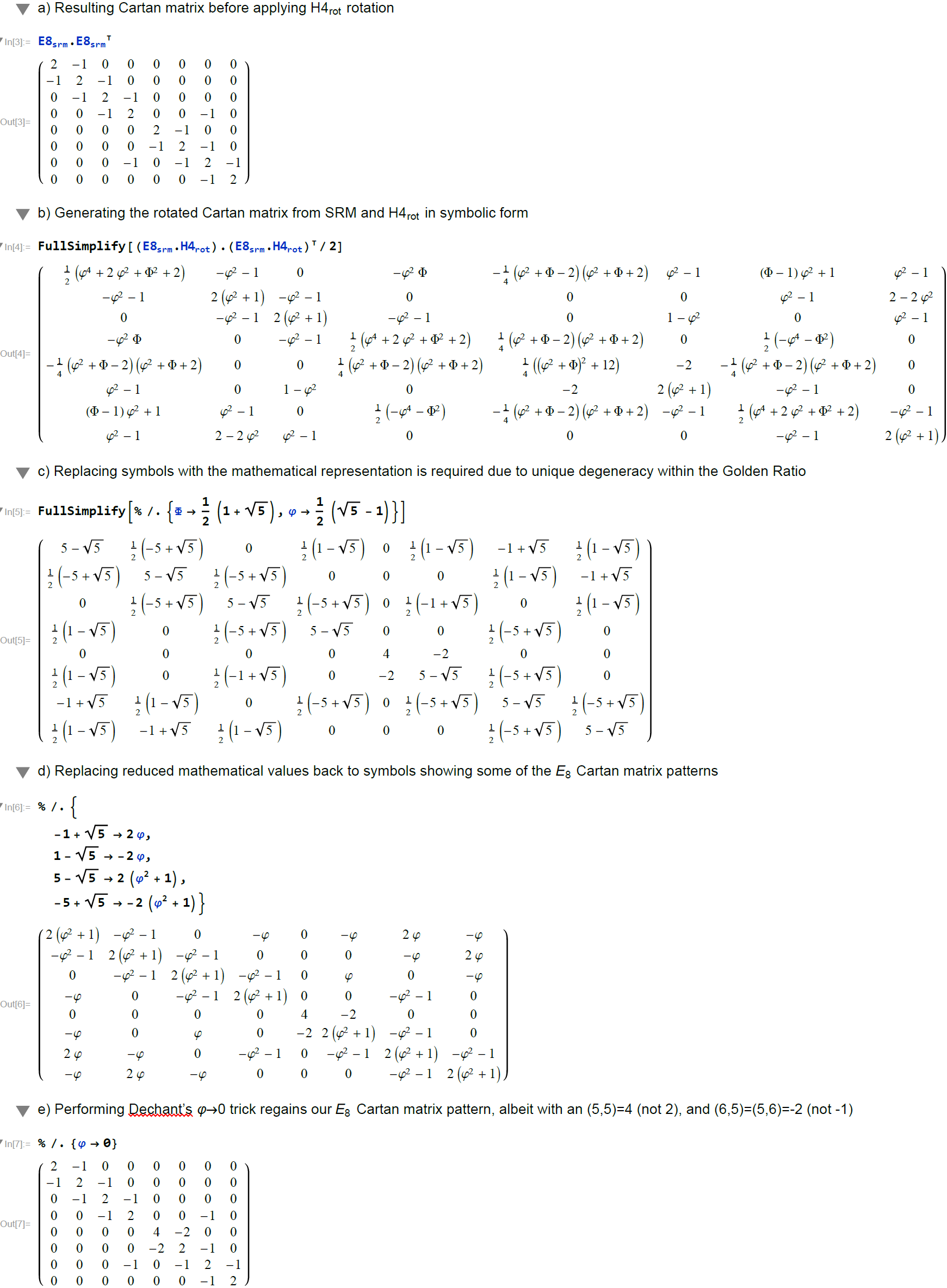FIG. 7: Symbolic analysis using MathematicaTM comparing the Cartan matrix before and after rotating the simple roots matrix (E8srm) used to create it (Note: the resulting Cartan matrix is not precisely that of E8, even after applying the Dechant’s Φ=0 trick)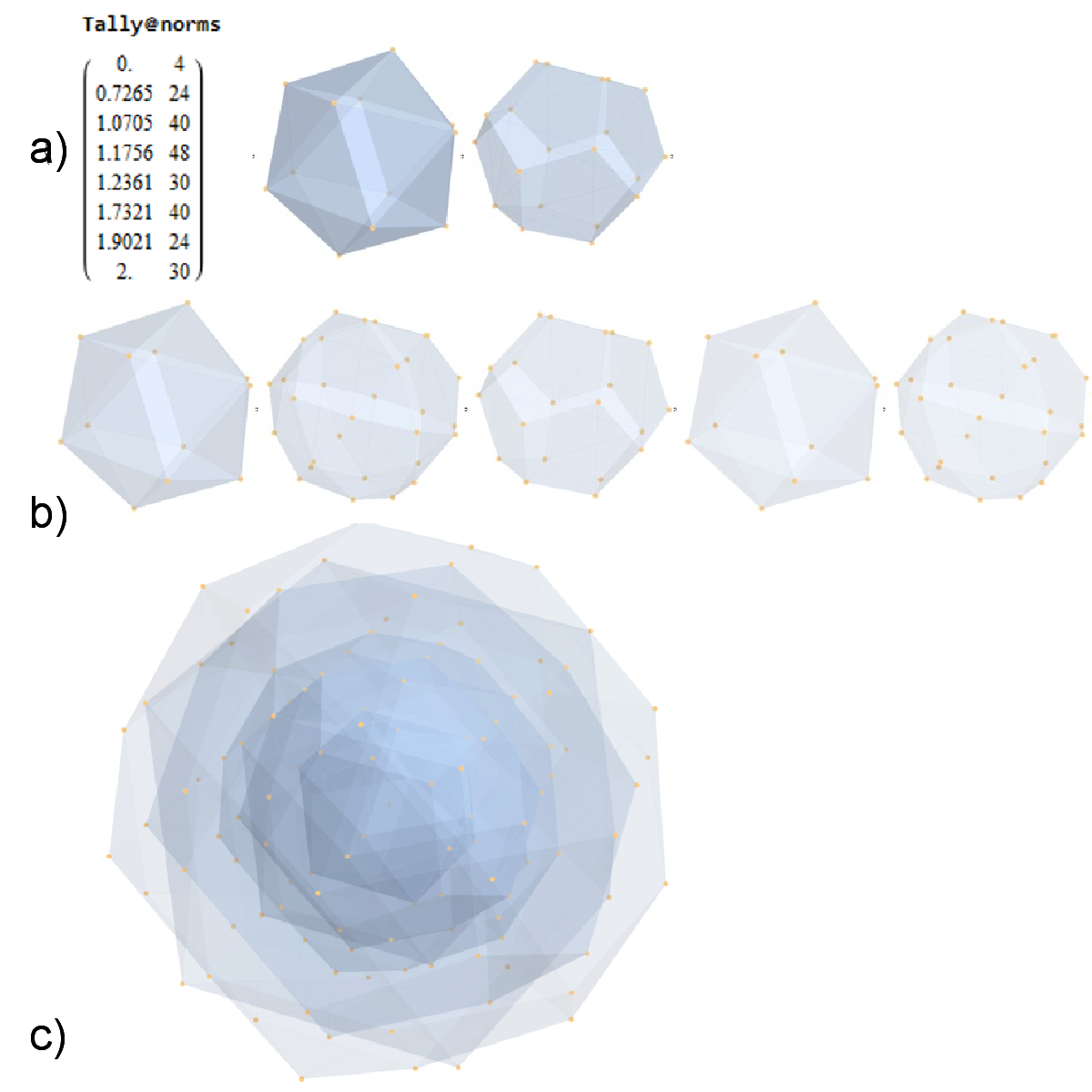FIG. 8: a) Sorted list of the vertex norms with their grouped vertex counts. b) 3D surface models for each of the 7 hulls of vertices c) Combined 3D surface model with increasing transparency for each successive hull.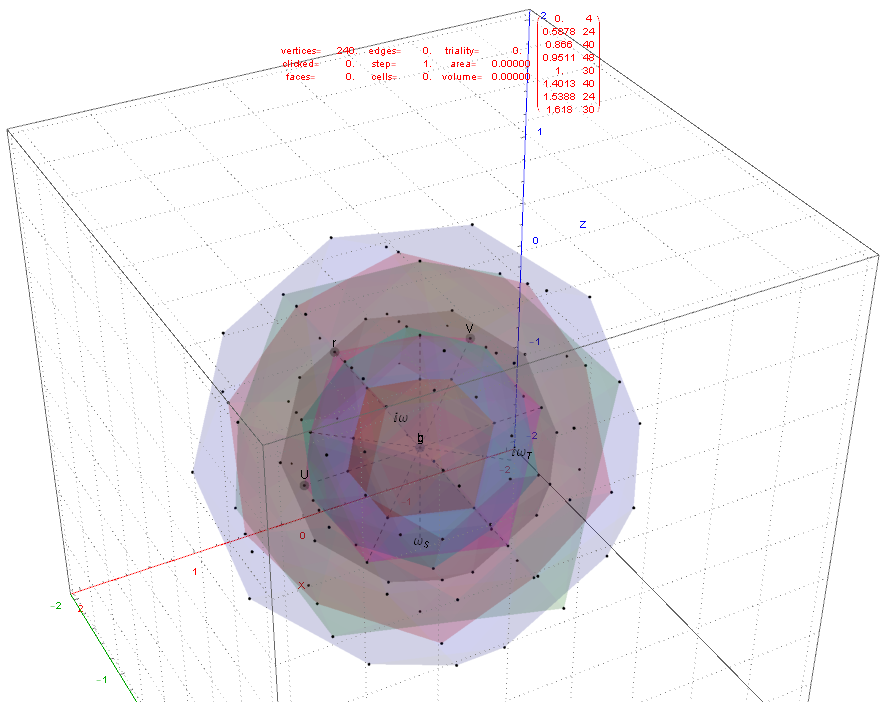FIG. 11: 3D projection using an orthonormal basis on H4 and H4Φ vertices (i.e. the rotated E8)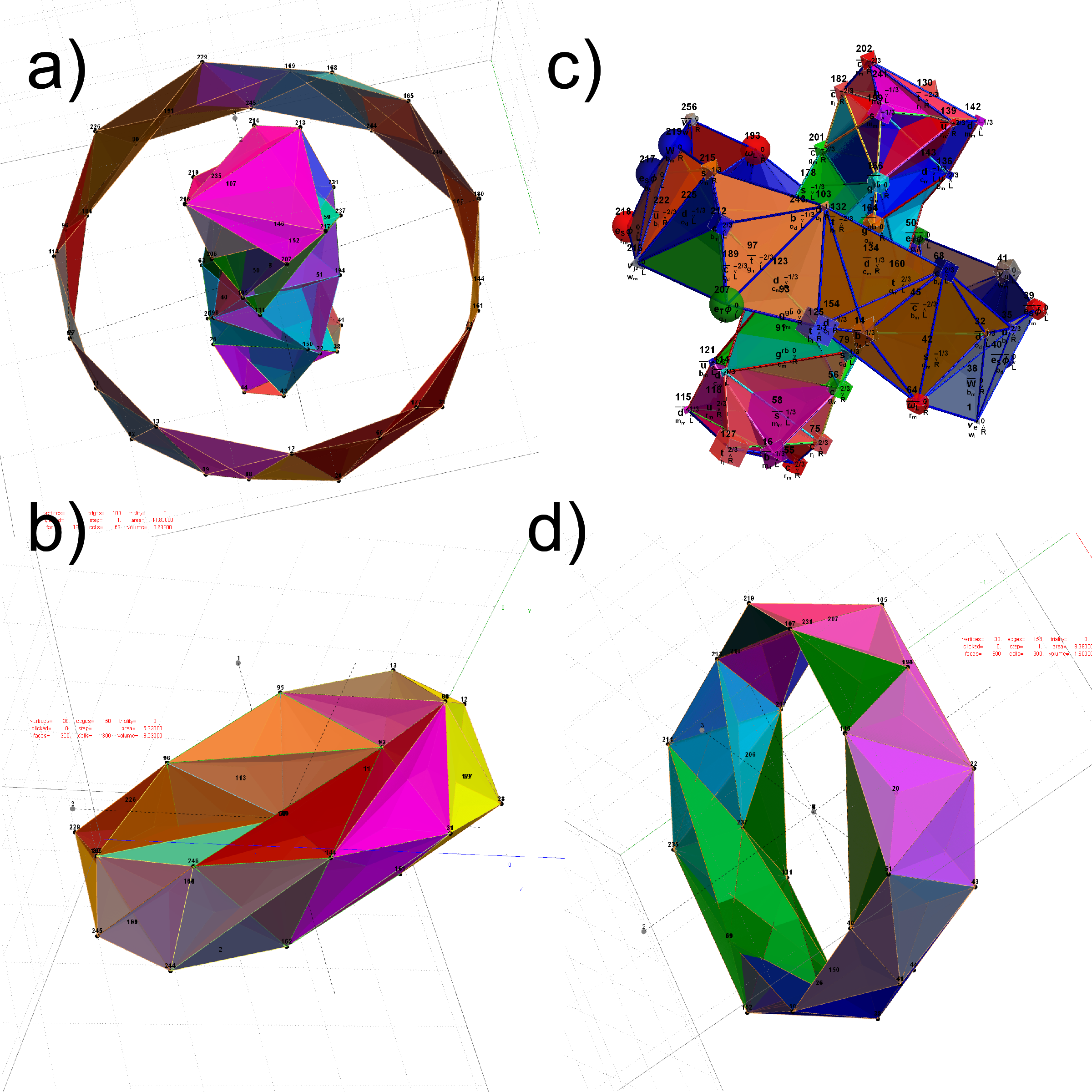FIG. 12: The Beordijk-Coxeter Tetrahelix emerging from the 8 concentric rings of 30 E8 and H4+H4Φ Petrie projection vertices; a) H4 inner and outer ring in 3D, b) with vertex size, shape, color assigned from physics model, c-d) inner and outer ring in orthonormal projection of H4Φ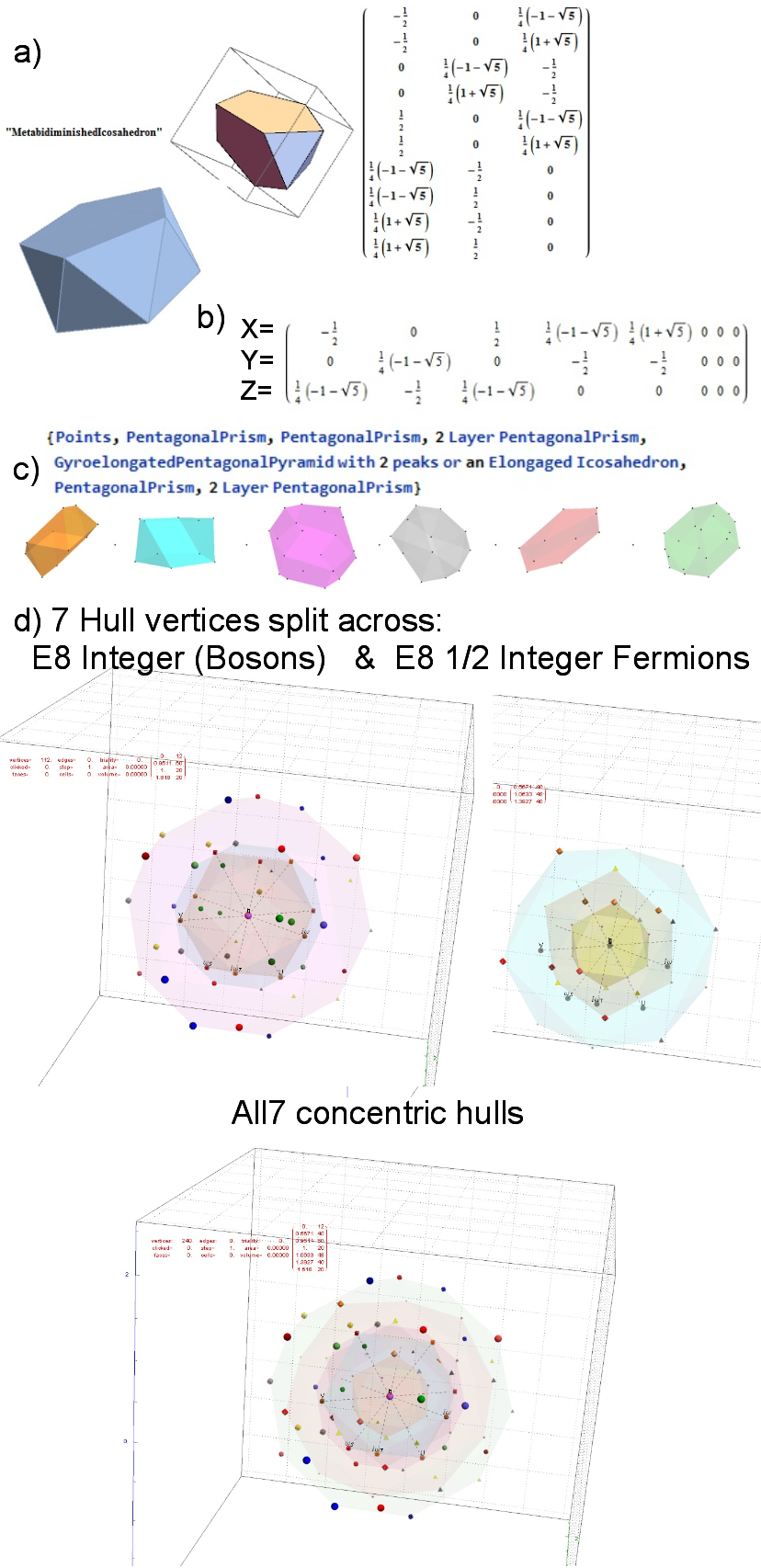FIG. 15: MetabidiminishedIcosahedron (with its negated vertices gives the GyroelongatedPentagonalPyramid) Crystal Projection Prism projecting E8 a) the crystal prism geometry and vertices, b) the selected basis vectors, c) the individual 3D projected concentric hull objects, d) the combined set of concentric objects with progressively increasing transparency and tally of vertex norms.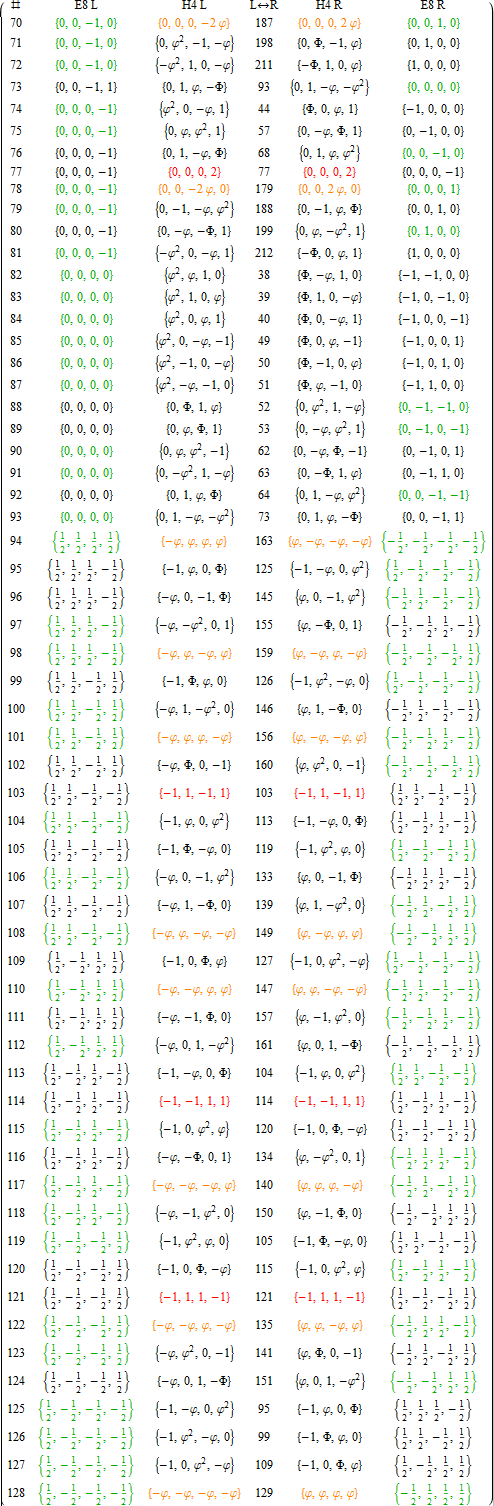APPENDIX A: LIST OF E8 AND ITS ROTATED H4 AND H4Φ VERTICES
This table splits the E8 and its rotated H4 vertices into L/R 4D elements.

1. Table structure
The first column is an SRE E8 vertex index number derived from sorting the E8 vertices by their position based on the 256=2^8 binary pattern from the 9th row of the Pascal triangle (1, 8, 28, 56, 70, 56, 28, 8, 1) and its associated Cl8 Clifford Algebra. This construction is described in more detail in . The odd groups (1,3,5,7,9) with (1,26,70,26,1) elements (respectively) are the 128 1/2 integer vertices. The even groups (2,4,6,8) with (8,56,56,8) elements (respectively) are the 112 integer vertices along with the 16 excluded 8 generator (and 8 anti-generator) vertices (2-9 and 248-255) with permutations of (1; 0; 0; 0; 0; 0; 0; 0) (a.k.a. the 8-Orthoplex), such that they indicate the basis vectors used for projecting the polytope.

Only the first half of the 240 vertices is shown, since the last half is simply the reverse order negation of the vertices
in the first half (e.g. the E8 vertex n=10 has as its’ negation 257-n=247). The middle column labeled L<->R indicates the vertex reference number that contains the same L as the R (and equivalently, the same R as the L, interestingly enough).

2. Table color coding
The E8 L/R columns’ green color-coded elements indicate that the 4D vertex rotates into the smaller H4 600-cell.
Conversely, the E8 L/R columns’ black color-coded elements indicate the 4D vertex rotates into the larger H4Φ
600-cell.

The H4 L/R columns’ red color-coded rows are 24-cell elements that always self-reference and are always members of the scaled up 600-cell H4Φ. The H4 L/R columns’ orange color-coded rows are 24-cell elements that always reference the negated elements in the range of 129-256 and are always members of the smaller 600-cell H4.

Metatron’s Cube

I was recently asked to create a 3D laser etched crystal of Metatron’s Cube.

The 13 Metatron rings are (or at least can be) taken from the 16 vertices of the tesseract (a 4D geometric figure called an 8-cell) which can be visualized by projecting 4D into 3D as two concentric 3D cubes.

Three of the 16 rings are hidden behind the ring in the front center when projecting the two concentric 3D cubes to 2D (or looking at it from just the correct angle on a corner and ignoring the perspective error introduced by parallax).

There are many more lines in the Metatron cube than from the typical 32 edges of the 8-cell tesseract connecting the vertices. These come from what is called the “complete graph” of 120 edges from the 16 vertices of the 8-cell. The Platonic solids are all contained (or related by duality) within the 3D projection of this complete graph of the 8-cell with large vertex spheres.

Slightly off angle (notice the 3 hidden spheres are now visible):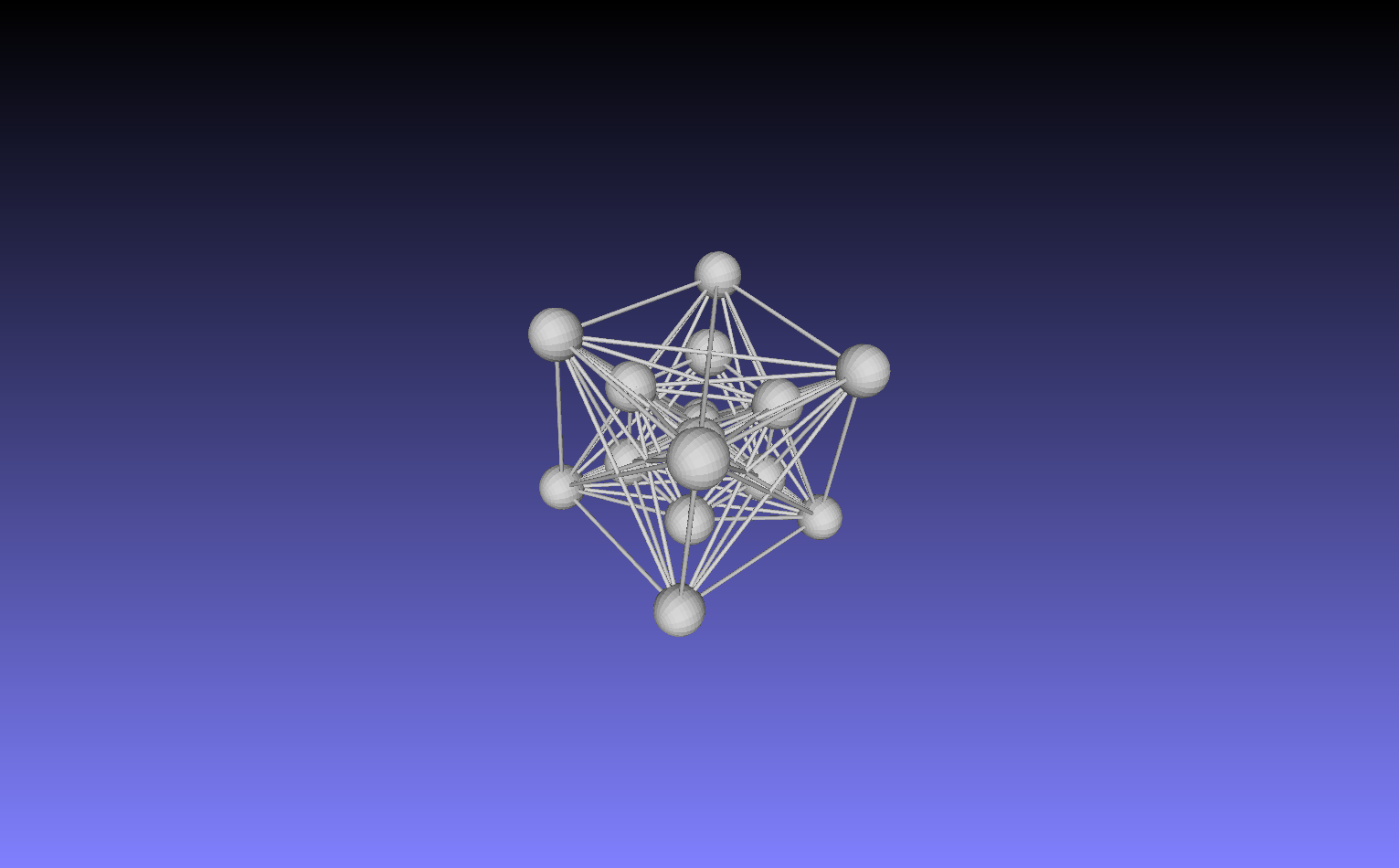E8 (2_41, 4_21, and 1_42) projected to various Coxeter planes

This is a shot of the relevant Mathematica code snippets that produce the images:E8 4_21 (with projection basis, edges, vertex overlap colors & counts) (click here for very large SVG):E8 2_41 (with projection basis, vertex overlap colors & counts) (click here for very large SVG):

A new ‘more Natural’ ToE model with Covariant Emergent Gravity as a solution to the dark sector

Please take a look at my latest paper based on my original work circa 1997-2007.
JGM/010-MOND-CEG-TOE [pdf, cite]
Title: A new ‘more Natural’ ToE model with Covariant Emergent Gravity as a solution to the dark sector
Authors: J Gregory Moxness
Comments: Apr 4 2018, 4 pages.
Subjects: General Relativity and Quantum Cosmology (gr-qc); High Energy Physics – Theory (hep-th)

For citations, remove the LaTex href tag structure if you don’t use the hyperref package.

3D Printed E7 1_32 Polytope Projected to the E6/F4 Coxeter Plane

The 3D model of the polytope: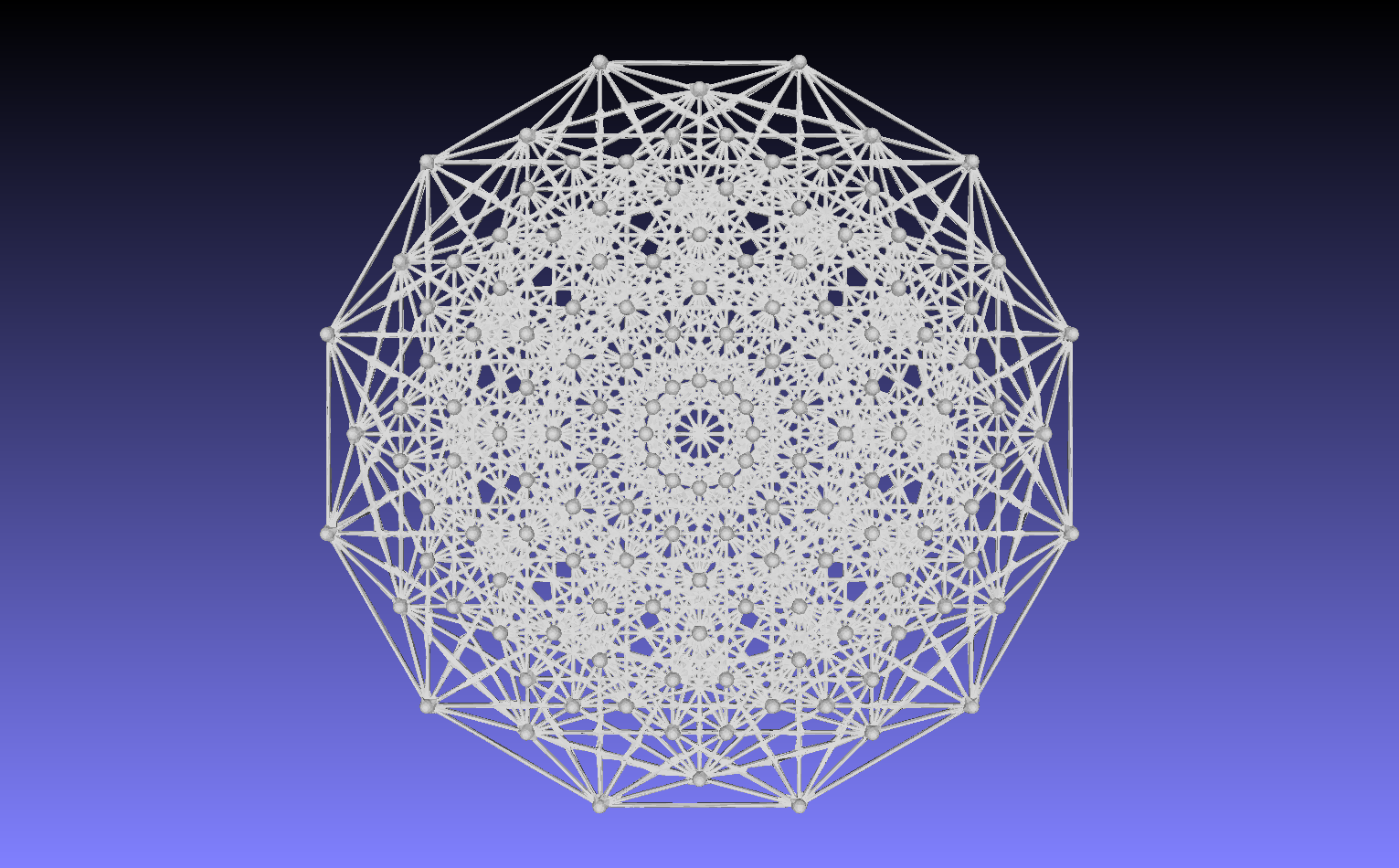3D printed in raw bronze:
Front: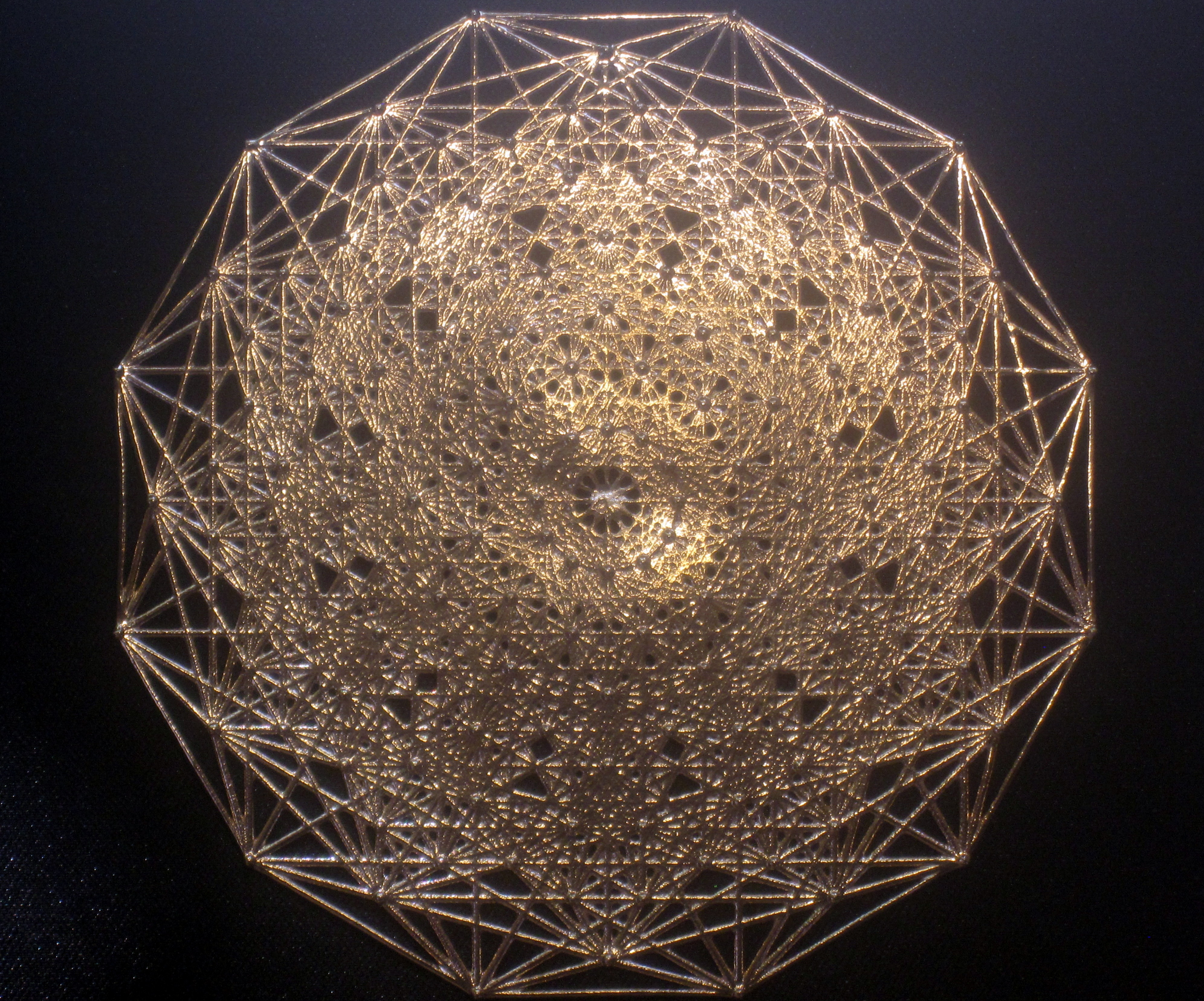Back:Here it is in a 3 1/8″x3 1/8″ laser etched optical crystal.G2, F4, E6, E7, and E8 triality relations in 3D concentric hulls of E8

Here are the G2 gluons connected by their trialities shown in a 3D concentric hull projection of E8 using the E8 to H4 folding matrix basis vectors. This is the 4th hull, which is the outer hull of the inner H4 600 cell (an icosadodecahedron). For more on E8 hulls, see this post.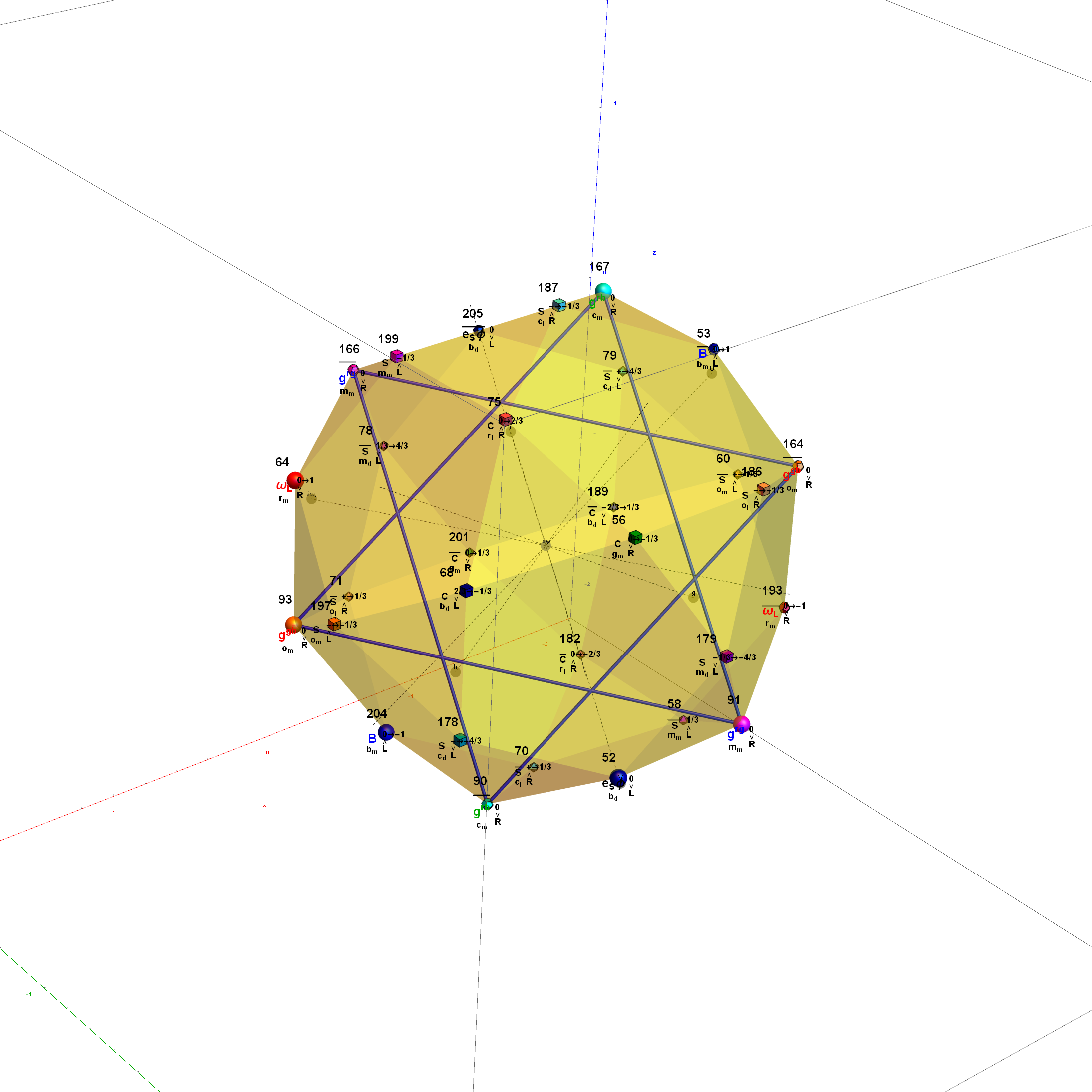The full F4 group with 10 T2 and 12 T4 trialities affecting the bosons is contained in the outer icosadodecahedron (1st hull) combined with the 3rd (quad icosahedral) and 4th icosadodecahedron hulls.

It is interesting to note that the two icosadodecahedron hulls comprise the 60 vertices of D6.Below is the full E8 with all trialities shown in 3D concentric hull projection.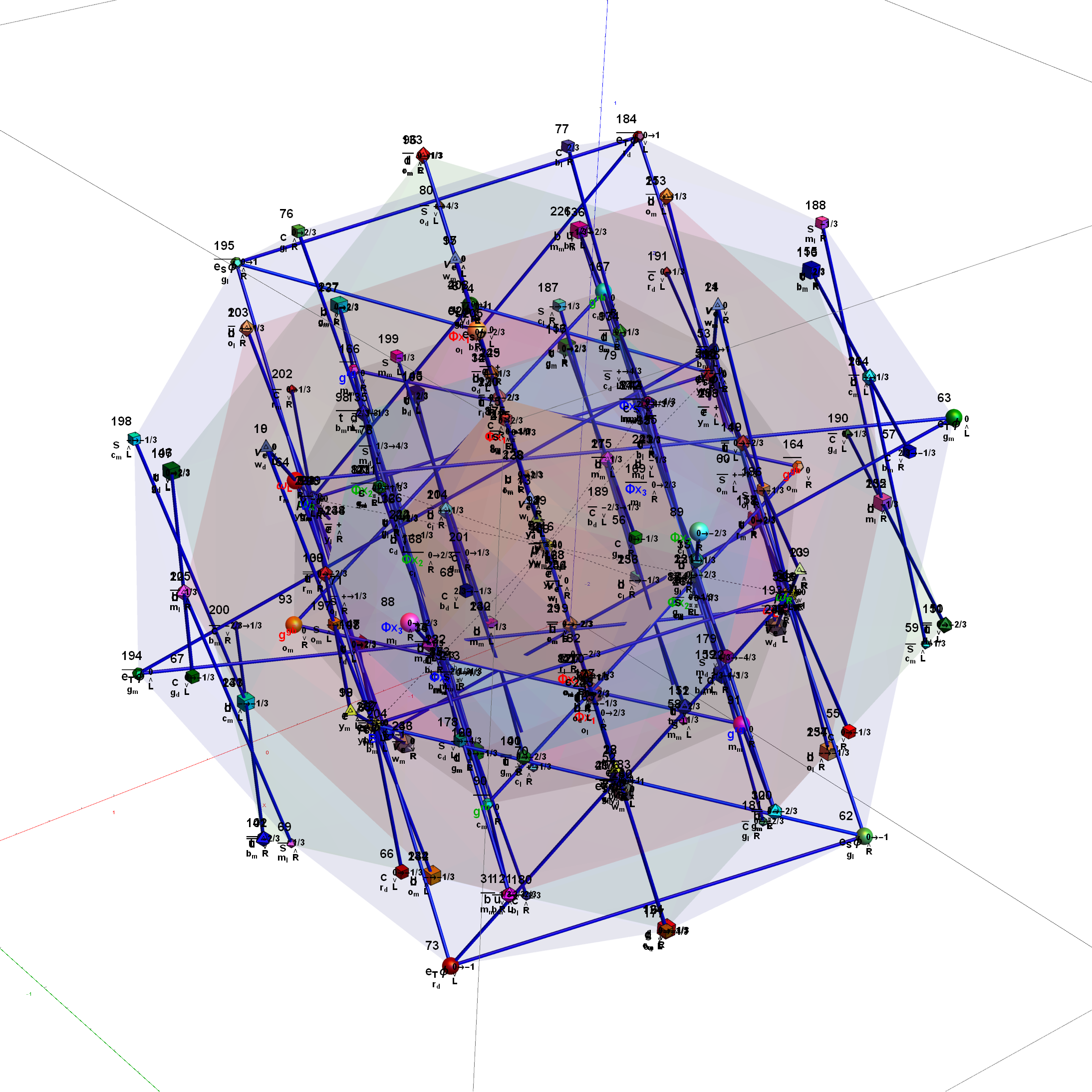Wow – that is nice! Remember, you heard it here first!

BTW – if you find this information useful, or provide any portion of it to others, PLEASE make sure you cite this post. If you feel a blog post citation would not be an acceptable form for academic research papers, I would be glad to clean it up and put it into LaTex format in order to provide it to arXiv (with your academic sponsorship) or Vixra. Just send me a note at: jgmoxness@theoryofeverthing.org.

Star of David Projection Basis for E8, E7 and E6

For a full discussion of the particle assignment symmetries involved, see http://vixra.org/pdf/1503.0190v1.pdf

E8 as 240 split real even vertices with  vertex color, size, shape, and labels from a modified A.G. Lisi particle assignment algorithm. The vertex number is the position in the Pascal Triangle (Clifford Algebra) representation of E8. The blue lines forming equilateral triangles represent the  10 Bosonic (or Color) Triality (T2) and 76 Fermionic (T4) rotations (using the 8×8 matrices shown below) that transform each member of the triangle into the next member.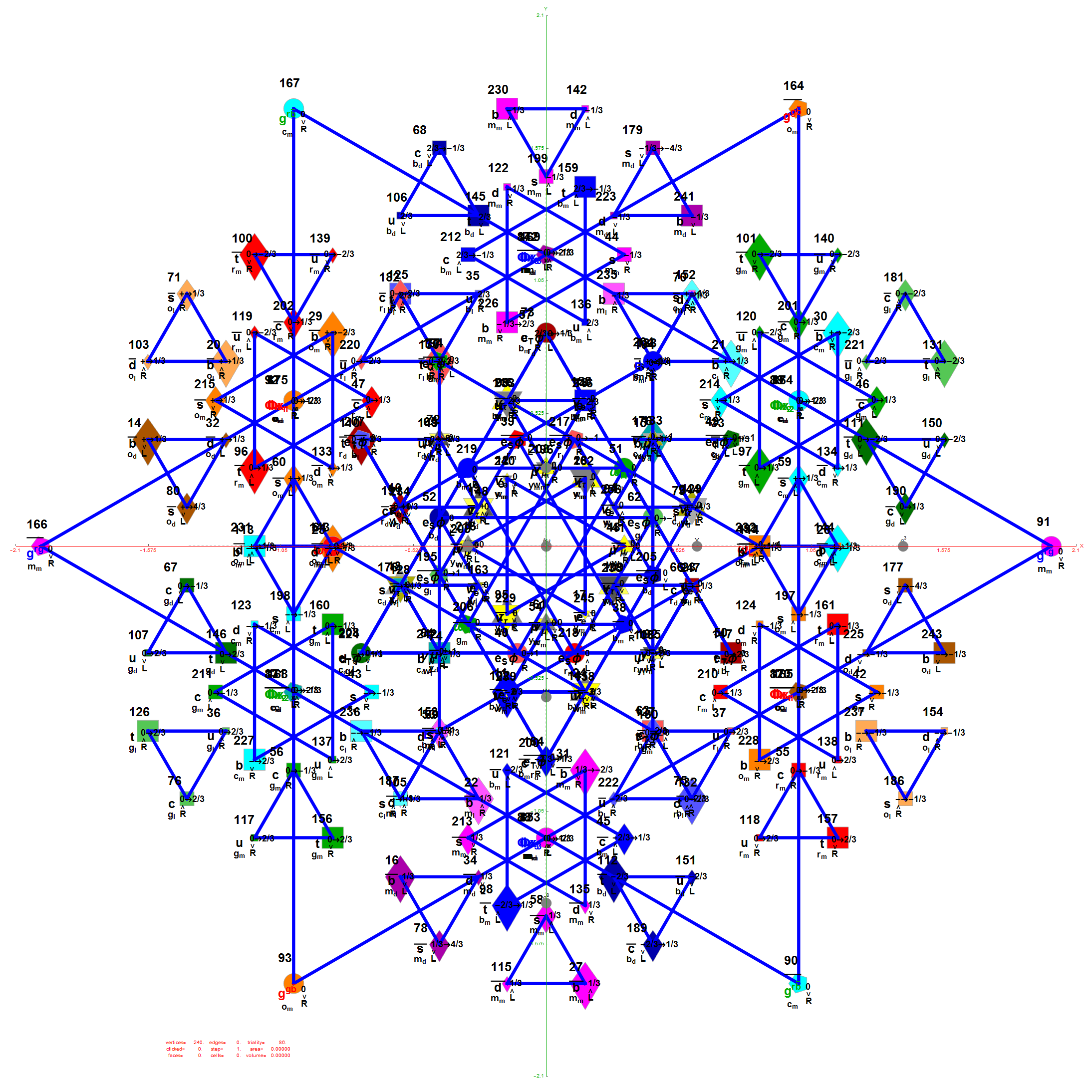E7 as derived from E8 above by taking the vertices of E8 with the last two columns being equal.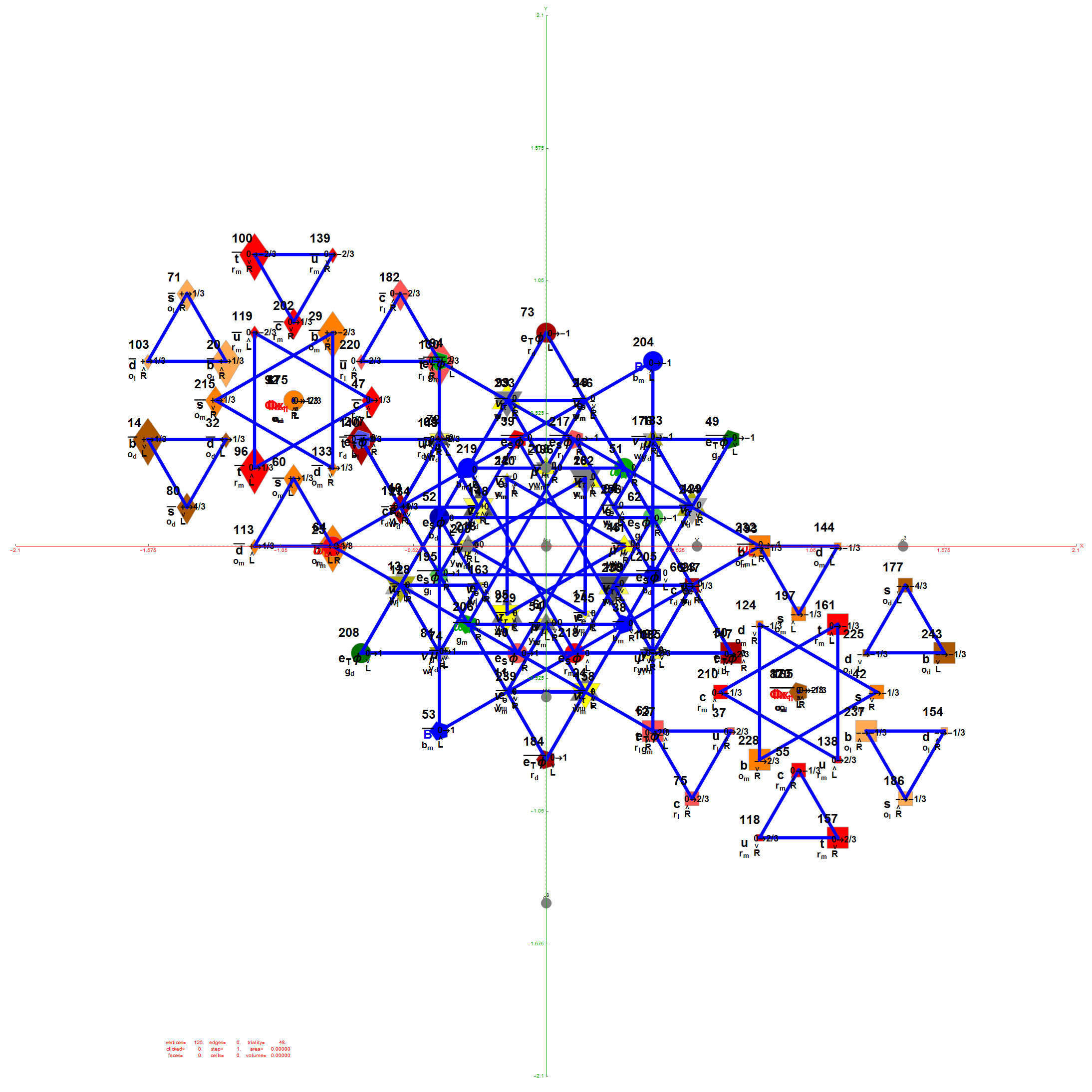E6 as derived from E8 above by taking the vertices of E8 with the last three columns being equal.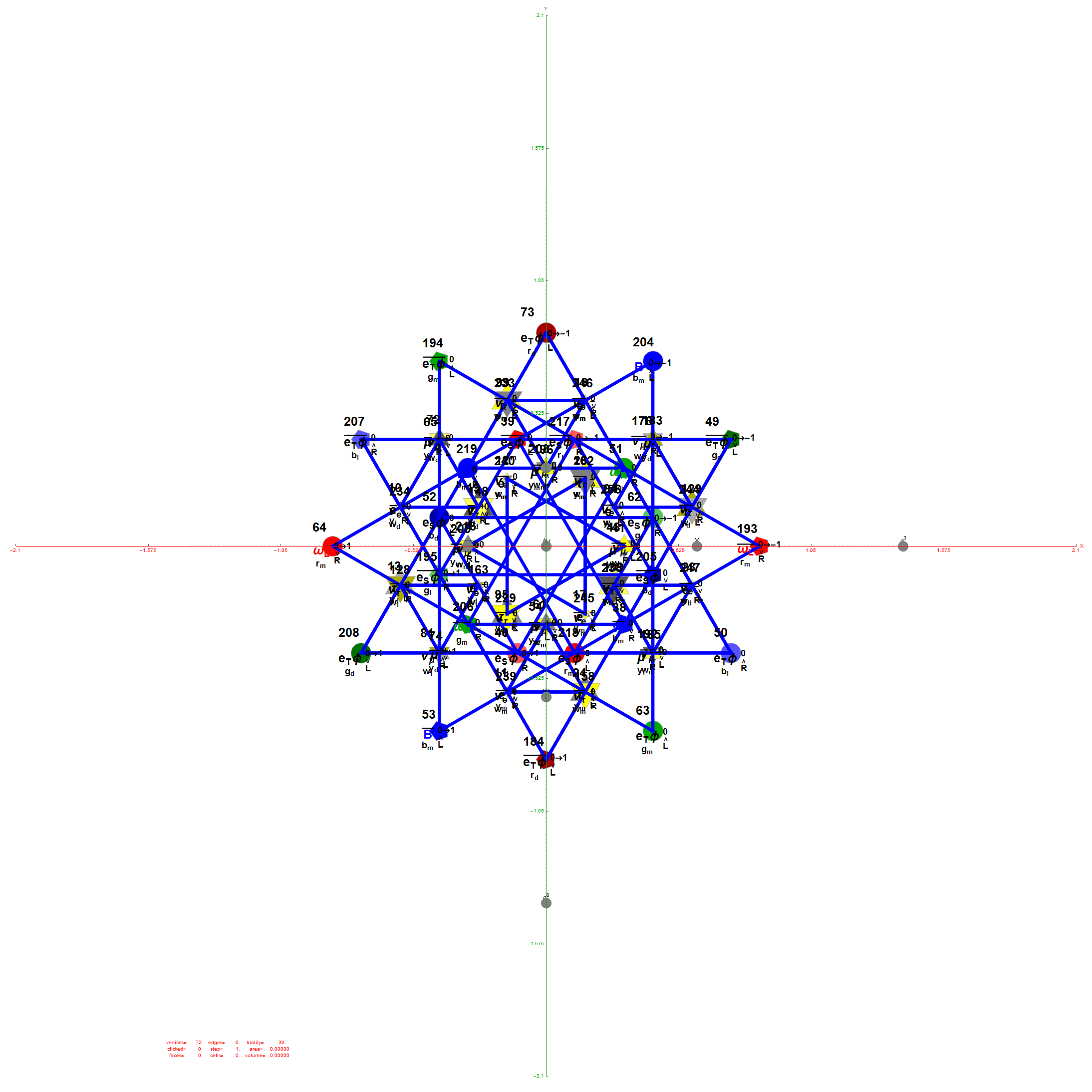The 48 vertices of F4 are made up of the 112 (D8) +/-1 integer pair vertices that are assigned to bosonic particles, which includes 22 trialities (10 from T2 and 12 from T4). The other 64 of 112  D8  vertices are assigned to the 2nd generation of Fermions. F4 is shown below with its sub-group G2 assigned to the gluons in the larger triality triangles.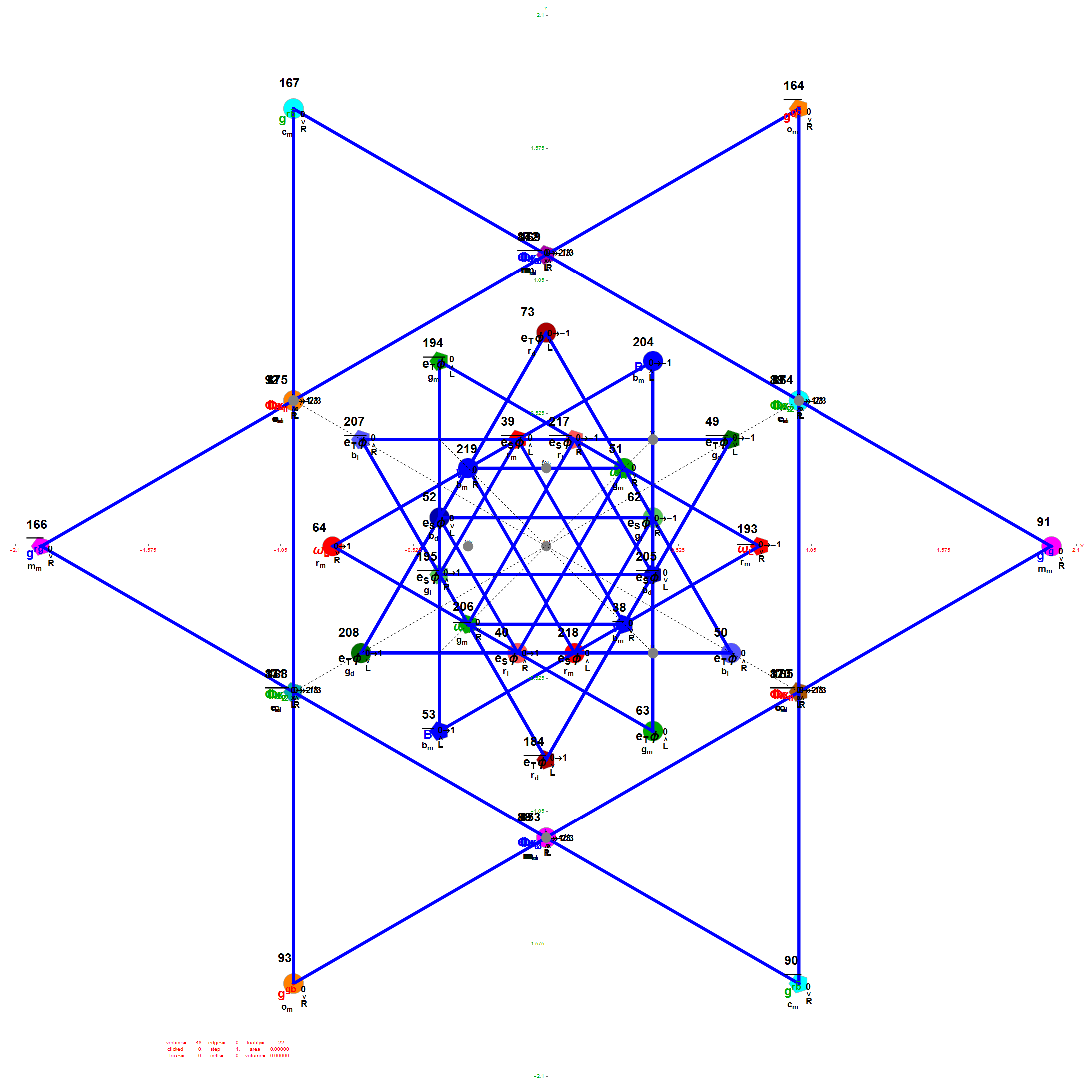Projection Basis for X and Y are H (first row) and V (second row) in the matrix below respectively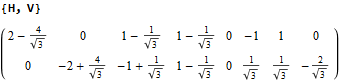The Triality Matrices are: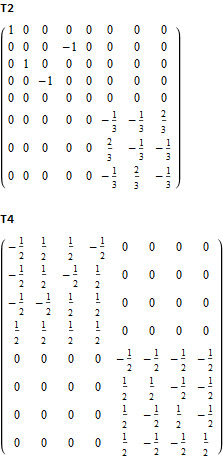The 10 Bosonic (Color) Triality Rotation Matrix (T2) equilateral triangles exclusively affect the particles that are derived from F4.

T2 rotates through the bosonic colors indicated by the last 3 columns of the E8 vertices appropriately labeled (r,g,b).The 76 Fermionic (Generation) Triality Rotation Matrix (T4) equilateral triangles. There are 64 fermion particle trialities that are derived from the 128 (BC8) half integer vertex assignments plus the 64 fermions assigned from the 112 (D8) +/-1 integer pair vertices. There is always one integer (2nd Generation) and two 1/2 integer vertices (1st and 3rd Generation) in every fermionic triality.

That is, T4 rotates through the fermionic generations.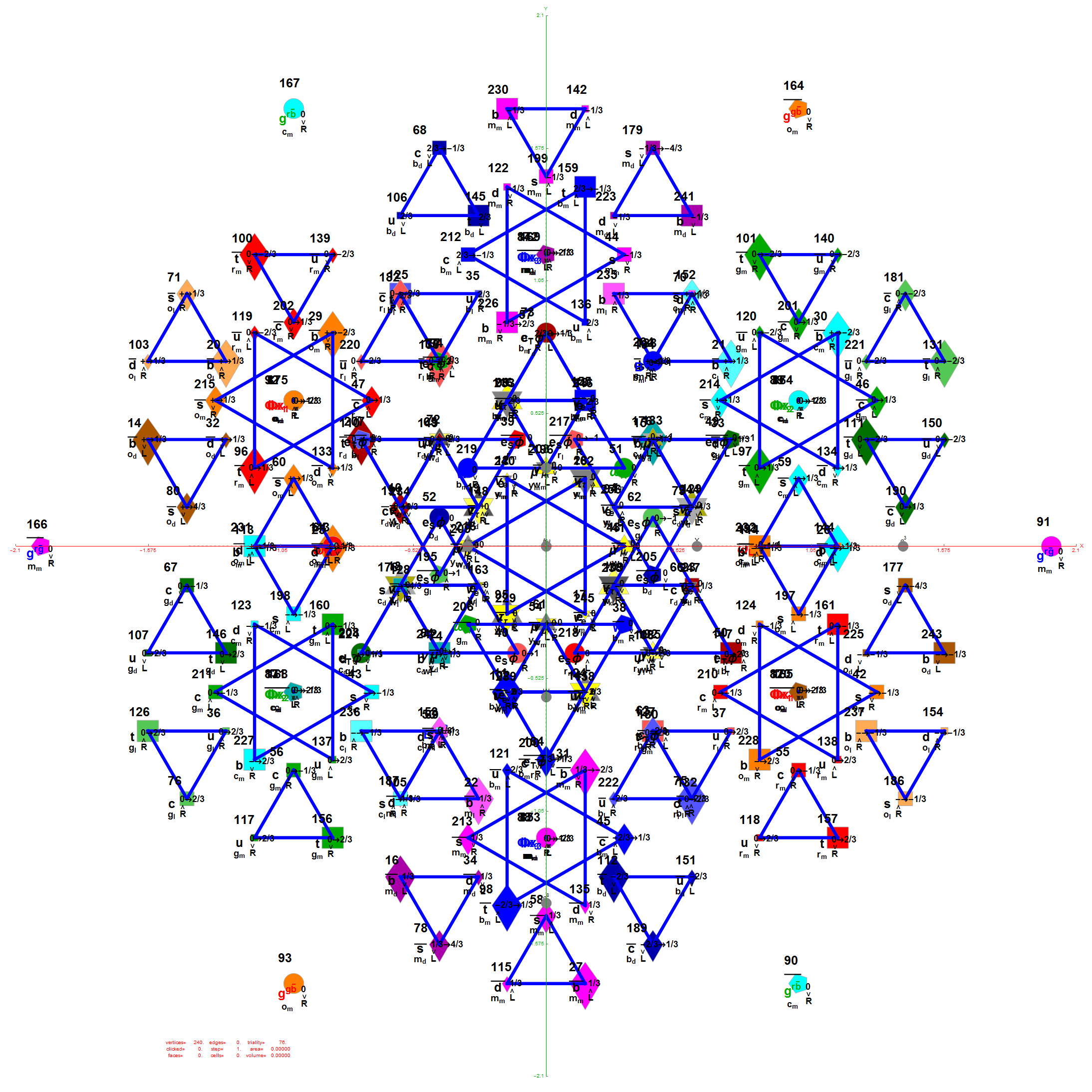There are 12 trialities from these 76 that are associated with the inner E6 & F4 Lie Group vertices assigned to bosons, shown below with only the 112 (D8) +/-1 integer pair unlabeled vertices: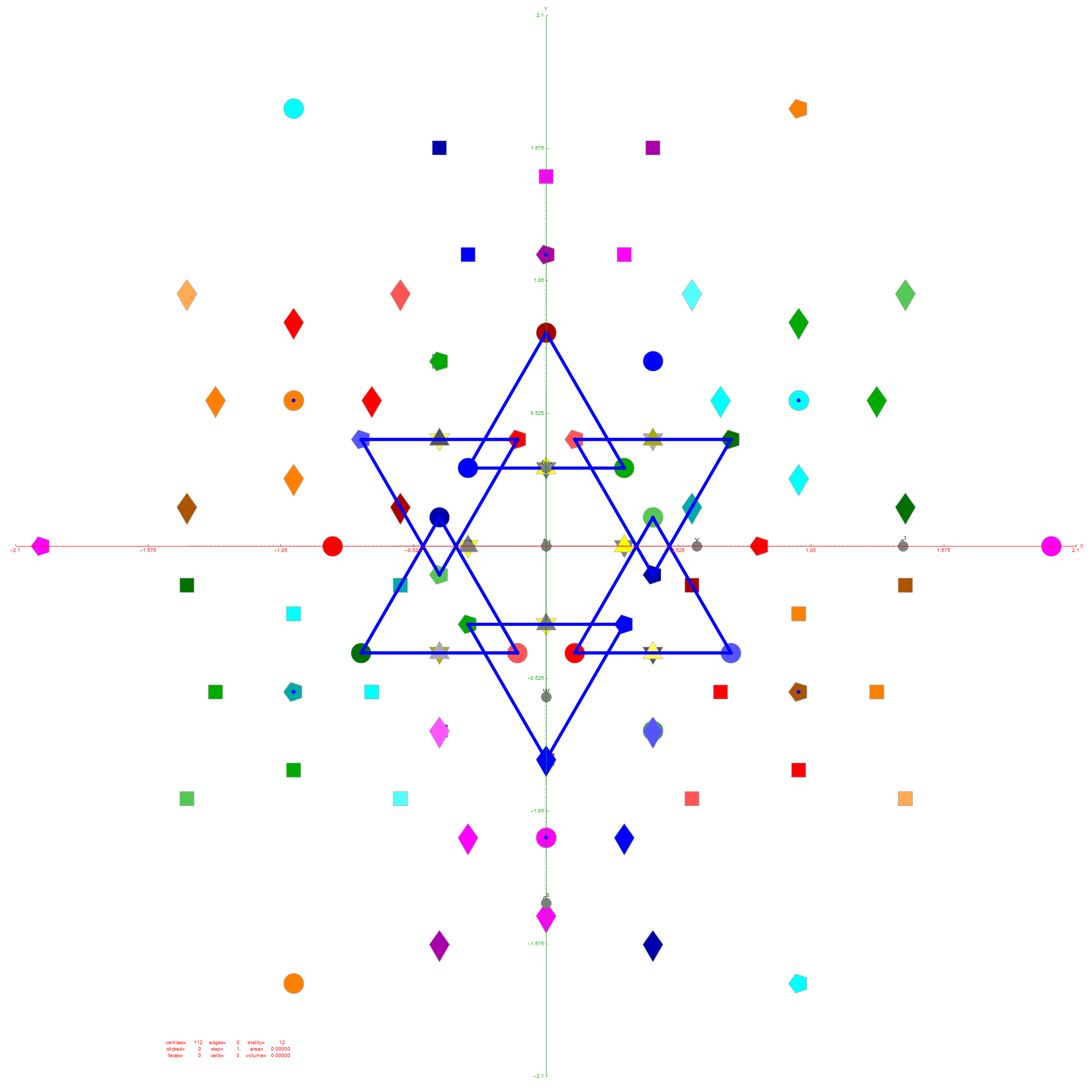Of course, there are a number of overlapping vertices in this projection stemming from the 112 (D8) +/-1 Bosonic sector. The following image colors the vertices by overlap count, with 120 Yellow with no overlaps, 24 Cyan with 2 overlaps (48), and 24 Cyan with 3 overlaps (72).

Notice the 8 projection basis vectors with dark gray circles. Of course, these projection basis vectors are the 8 “generator vertices” of E8 vertices with permutations of {+1,0,0,0,0,0,0,0} giving it the full 240+8=248 dimension count. Add to that the -1 “anti-generators” being the opposite end of the projection basis vectors and you get the full 256 vertices representing the full Clifford Algebra on the 9th row of the Pascal Triangle.There are 6720 edges in E8 with an 8D length Sqrt.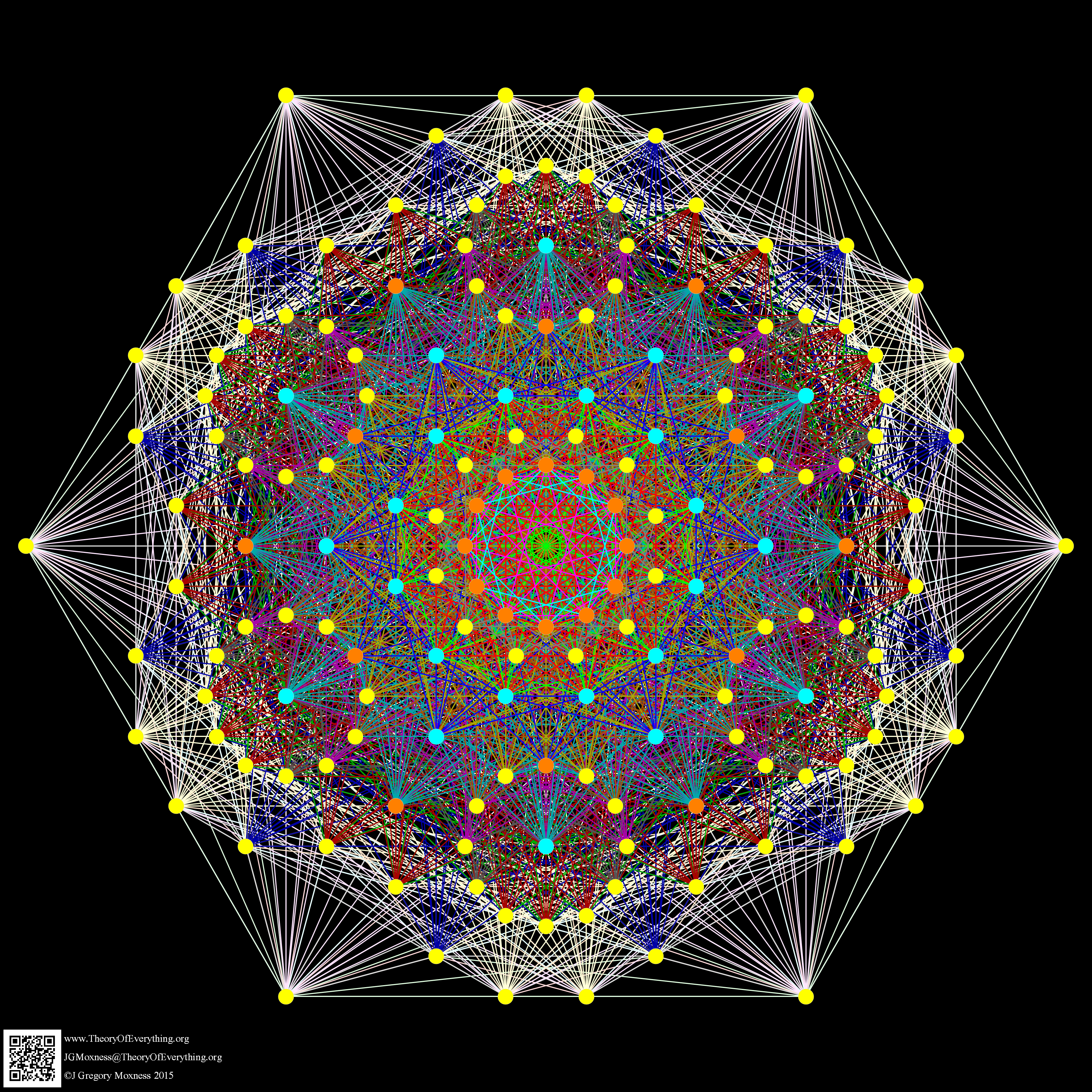Just for fun, I introduce to you the 6720 rectified E8 vertices taken from the midpoint of each edge and using the same projection with coloring of the spheres based on overlap count. Enjoy!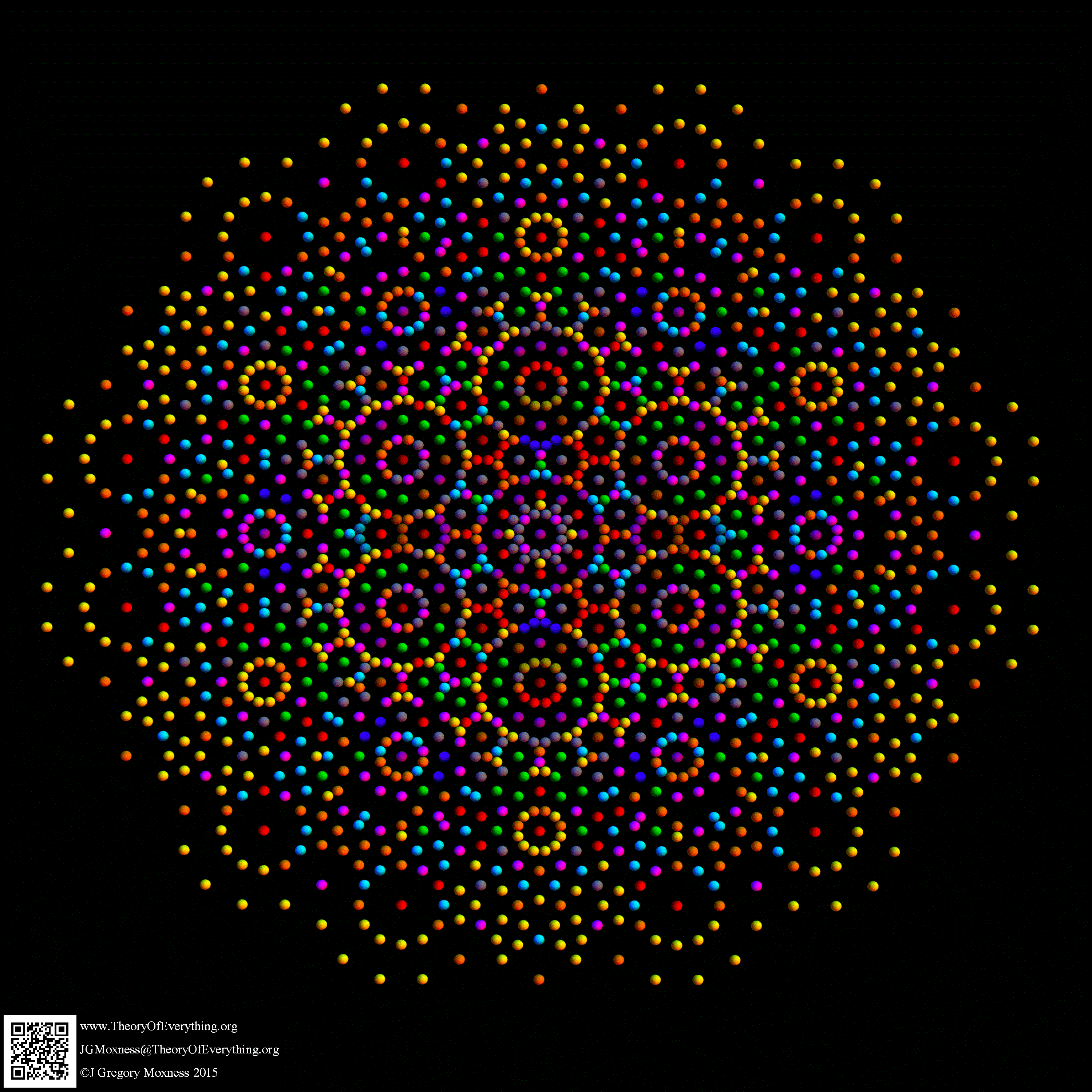Here are the G2 gluons connected by their trialities shown in a 3D concentric hull projection of E8 using the E8 to H4 folding matrix basis vectors. This is the 4th hull, which is the outer hull of the inner H4 600 cell (an icosadodecahedron). For more on E8 hulls, see this post.The full F4 group with 10 T2 and 12 T4 trialities affecting the bosons is contained in the outer icosadodecahedron (1st hull) combined with the 3rd (quad icosahedral) and 4th icosadodecahedron hulls.Below is the full E8 with all trialities shown in 3D concentric hull projection.Wow – that is nice! Remember, you heard it here first!

BTW – if you find this information useful, or provide any portion of it to others, PLEASE make sure you cite this post. If you feel a blog post citation would not be an acceptable form for academic research papers, I would be glad to clean it up and put it into LaTex format in order to provide it to arXiv (with your academic sponsorship) or Vixra. Just send me a note at:  jgmoxness@theoryofeverthing.org.